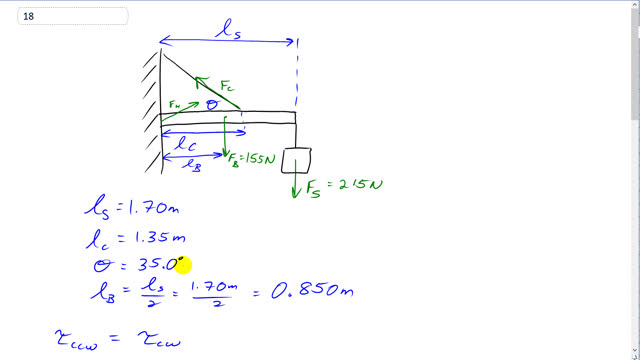## You are here

Question:

A shop sign weighing 215 N hangs from the end of a uniform 155-N beam as shown in Fig. 9–58. Find the tension in the supporting wire (at $35.0 ^\circ$), and the horizontal and vertical forces exerted by the hinge on the beam at the wall. [Hint: First draw a free-body diagram.]Problem 9-58.

Giancoli, Douglas C., Physics: Principles with Applications, 7th Ed., ©2014. Reprinted by permission of Pearson Education Inc., New York.
The question will be visible after logging in, as required by Pearson Education Inc.

$F_c = 643 \textrm{ N, } F_{Hx} = 526\textrm{ N, } F_{Hy} = 2 \textrm{ N}$